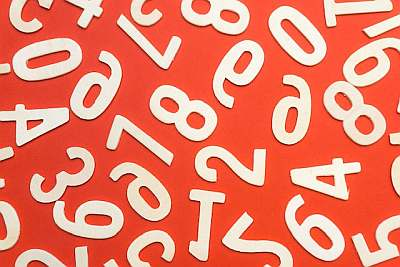# Fraction to Percentage Calculator

Instructions: Use this step-by-step fraction to percentage calculator to compute what percentage is associated to a fraction D/X. Please provide the required information in the form below:The fraction $$D / X$$ (Ex: 2/3, etc) =

You will compute the percentage associated to the given fraction $$D / X$$.

## Fraction to Percent Calculator

The idea of a fraction and a percentage are tightly related. Indeed, a percentage is, by its name, is how much of a total the value is, if the total value were 100.

So, it is like amplifying the terms in the fraction so that the denominator has 100 in it. The resulting value in the numerator is the percentage. But there must be an easier way of seeing it. Well, you bet!

### How Do You Compute a Fraction into a Percentage

So you want to know how to convert fraction to percentage without calculator, right? We compute the required percentage associated to a fraction by simply using the following formula:

$\text{Percentage} = \text{Decimal expression of } \displaystyle\frac{D}{X} \times 100$

That is all. You take the decimal expression of the fraction $$\frac{D}{X}$$, and then, the result of that decimal expression is multiplied by 100, and you get your desired percentage.

### Example of converting a fraction into a percent

Example: What percentage is 13 of 38?

Using the above formula, we get:

$\text{Percentage} = \displaystyle\frac{13}{38} \times 100$ $= 0.342105263 \times 100$ $= 34.21 \%$

### Another fraction to percent example

Write 2/5 as a percent?

Solution: Notice that

$\displaystyle\frac{2}{5} = \displaystyle\frac{4}{10} = 0.4$

which can be converted as a percent using the formula

$0.4 \times 100 = 40%$

Hence, 2/5 corresponds to 40%### Pros and cons of using decimals versus percentages

We know decimals and percentages are closely related. Indeed, you get a decimal, multiply by 100 and you get the corresponding percentage. But then, which one should I use?

• Using numbers or percentages is highly contextual, and will depend on what you are using it for
• In the context of using arithmetic operations, it will be more practical to use decimals as opposed to percentages
• When dealing with finance and money subjects, likely percentages will be more interpretable
• Often times it will absolutely clear what to use, but you can always easily convert between decimals and percentages, if the need arises

### Is This Related to a Regular Percentage Calculator?

Yes, absolutely, the idea of converting a fraction to percentage is tightly related with the concept of computing a regular percentage . They are essentially the same thing. When you are computing the percentage corresponding to the fraction $$\frac{D}{X}$$, what you are really doing is to compute what percentage $$D$$ is of $$X$$.

This is also tightly related with the conversion of a fraction into a decimal, considering that we know that there is a tight association between decimals and percentages.

Indeed, you can try this decimal to percent calculator which will show step-by-step the mechanics of the conversion, or reversely you may be interested in converting a percent to decimal.